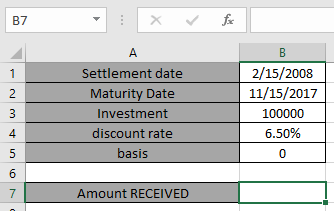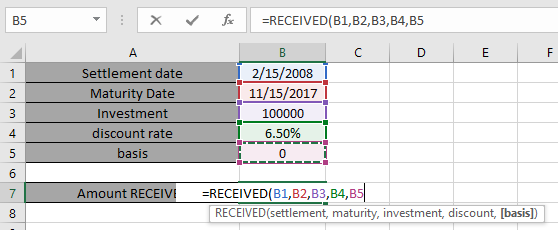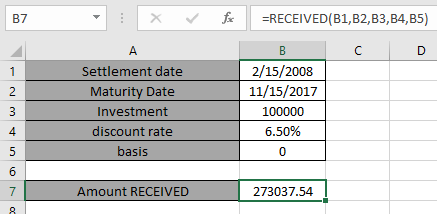# How to use the RECEIVED Function in Excel

In this article, we will learn about how to use the RECEIVED function in Excel.
RECEIVED function calculates the amount which is received at maturity for a bond with an initial investment (security) and a discount rate, there are no periodic interest payments.

RECEIVED = investment / ( 1 - discount * DIM/B)

Here B is the number of days in a year, depending on the year basis & DIM is the number of days from issue of maturity.

The RECEIVED function is a built-in financial function which returns the amount RECEIVED at maturity for a fully invested security.

Syntax:

=RECEIVED (settlement, maturity, investment, discount, [basis])

Settlement : Settlement date of the security
Maturity : Maturity date of the security. The maturity date is the date when the security expires.
Investment : amount invested in the security
discount : The security's discount rate
basis : [optional] Day count basis. Default is 0.

 Basis Day count basis 0 US (NASD) 30 / 360 1 actual / actual 2 actual / 360 3 actual / 365 4 European 30 / 360

Let’s understand this function using it in an example.Here we have values to calculate the amount received at maturity with an initial investment (security) and a discount rate for a bond having a settlement date & maturity date.

Use the formula:

=RECEIVED(B1, B2, B3, B4, B5)

B1 : Settlement date of the security
B2 : Maturity date of the security. The maturity date is the date when the security expires.
B3 : investment amount in the security
B4 : The security's annual coupon rate
B5 : 0 specifies Day count basis method choose US (NASD) 30 / 360 .[optional]Here all the arguments to the function is given as cell reference.

Note: Dates in the formula must be entered using cell reference or DATE function. Excel date format creates #VALUE! error while computing results. Use the cell reference or DATE function in excel while taking date as input to the function
Now Press Enter to get the RECEIVED amount at maturityThe RECEIVED amount is 273,037.54

Note:

1. Excel stores dates as serial number and is used in calculation by the function. So it’s recommended to use dates as cell reference instead of giving direct argument to the function.
2. Arguments settlement , maturity & basis are truncated to integers.
3. The function returns the #VALUE! Error if settlement date & maturity date is not a valid date format.
4. The function returns the #NUM! Error if:
1. Investment is less than or equals to zero. Investment 0.
2. Discount is less than or equals to zero. discount 0.
3. If value of argument basis is either < 0 or  > 4.
4. If maturity settlement.

Hope you understood how to use RECEIVED function and referring cell in Excel. Explore more articles on Excel function here. Please feel free to state your query or feedback for the above article.

Terms and Conditions of use

The applications/code on this site are distributed as is and without warranties or liability. In no event shall the owner of the copyrights, or the authors of the applications/code be liable for any loss of profit, any problems or any damage resulting from the use or evaluation of the applications/code.